# IB DP Physics: HL复习笔记7.1.9 Investigating Half-Life

### Investigating Half-Life

#### Aim of the Experiment

• The aim of the experiment is to investigate the half-life of a decaying radioactive sample
• This can be done with a live sample if safe access is available
• This can also be done through an online simulation

Variables

• Independent variable = time, t
• Dependent variable = radioactive activity, A
• Control variables:
• Size of sample
• Same distance from detector to sample
• Same material for sample

#### Equipment List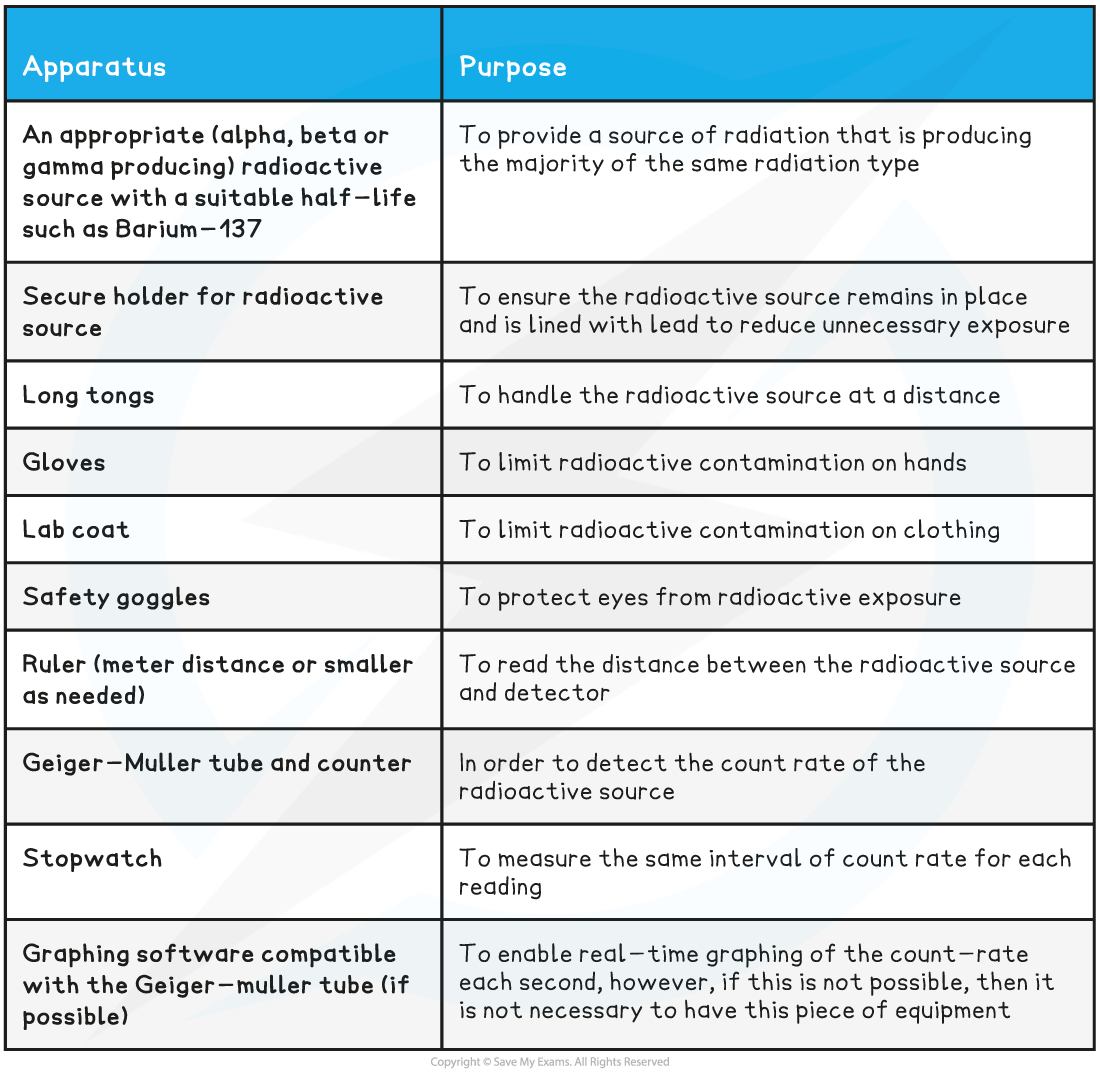• Resolution of equipment:
• Metre ruler = 1 mm
• Stopwatch = 0.01 s

#### Method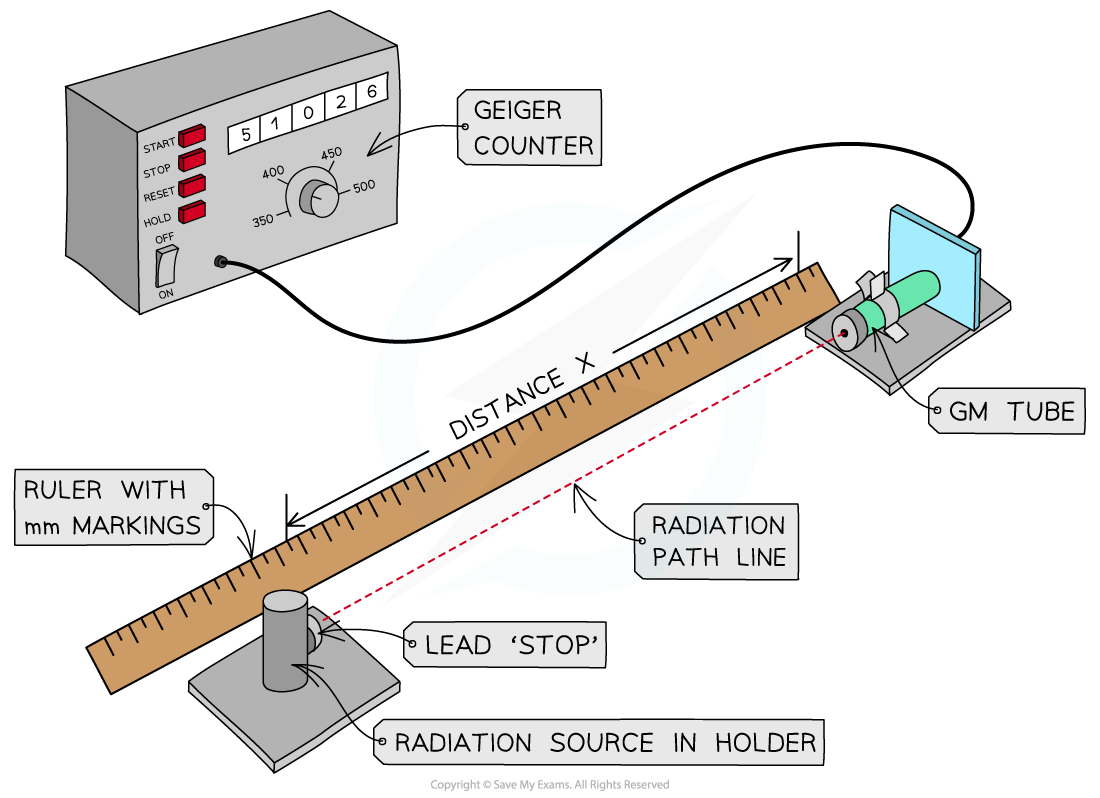1. Measure the background radiation for 30 second intervals using a Geiger-Muller tube without the radiation source in the room, take several readings and find an average
2. Next, put the radiation source at a set starting distance appropriate for the ionizing strength of the radiation (e.g. 1 - 2 cm for an alpha source, 2 - 5 cm for a beta source and 15 - 30 cm for a gamma source) from the GM tube
3. If graphing software is available to use with the Geiger-Muller tube, then that should be started as soon as the source is placed in position, if not, then proceed to step 4
4. Once the sample is in place measure the number of counts in 30-second intervals for 10 minutes or until the activity greatly decreases from the beginning value
5. Remove sample and replace into a lead-lined box or safe storage container
6. Repeat this for several trials if possible using fresh same size samples

A suitable table of results might look like this: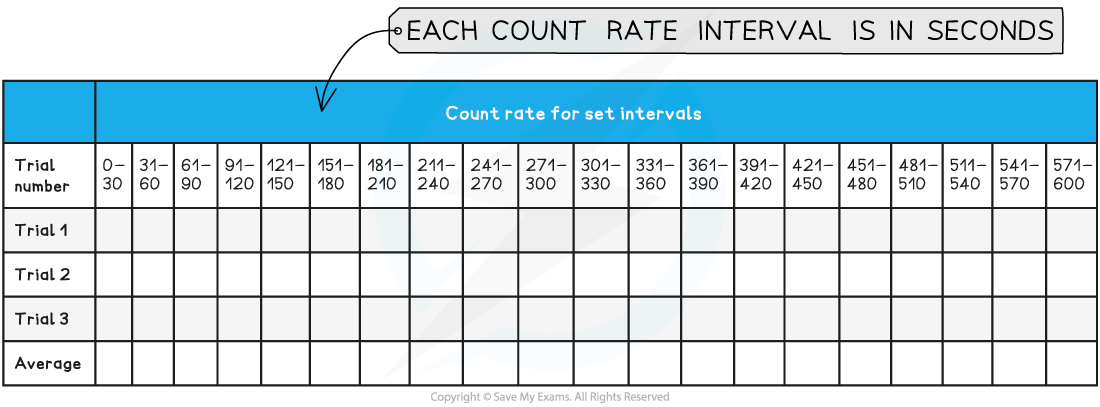#### Analysis of Results

• What is important for this experiment is the initial count rate and the following decrease as the radioactive source decays from the parent nuclei into the daughter nuclei.
• The background rate must be subtracted from the data before analysis can begin. This is true for both graphed and manual data
• In order to see the decrease and model it, the data should be graphed with the correct count rate (background subtracted) on the y-axis and the time intervals on the x-axis. If graphing software is used then the graph should look similar to the following: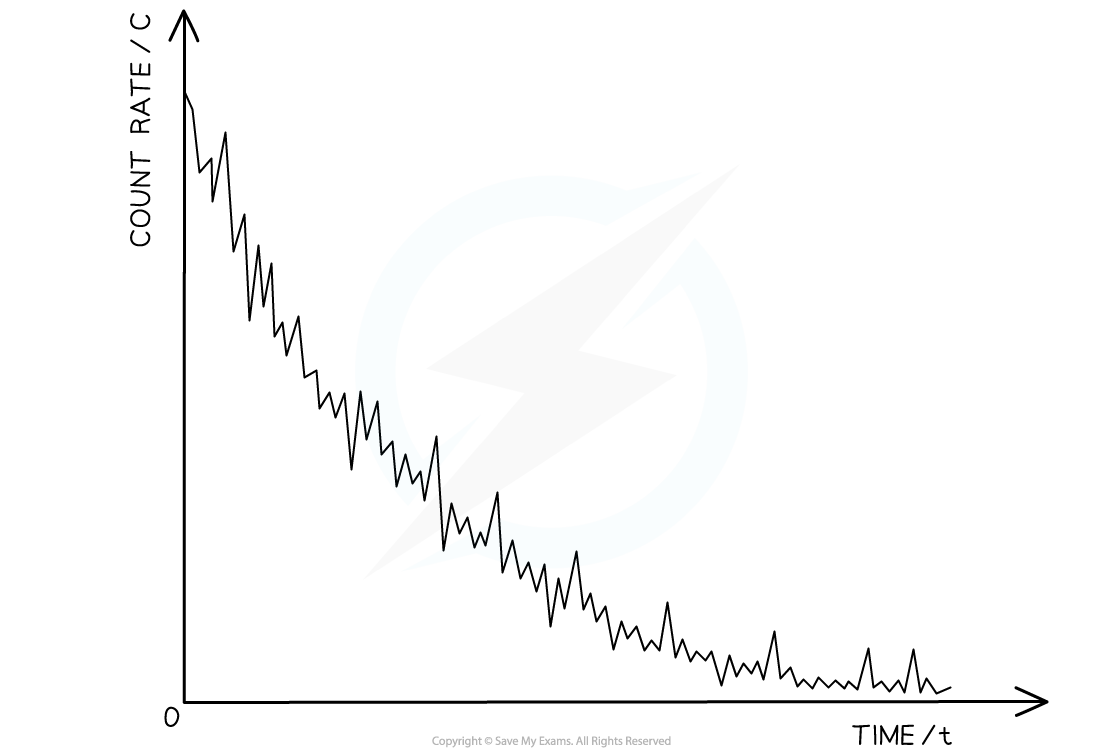• However, if the manual method is used, then there will be twenty data points rather than a smooth curve with fluctuations
• Using this curve, the half-life can be found by comparing the initial magnitude of the activity with the time it takes to decay to half of the original activity.
• This can be extended by finding the time taken for two half-lives to pass and the activity to reach one quarter for quantitative comparison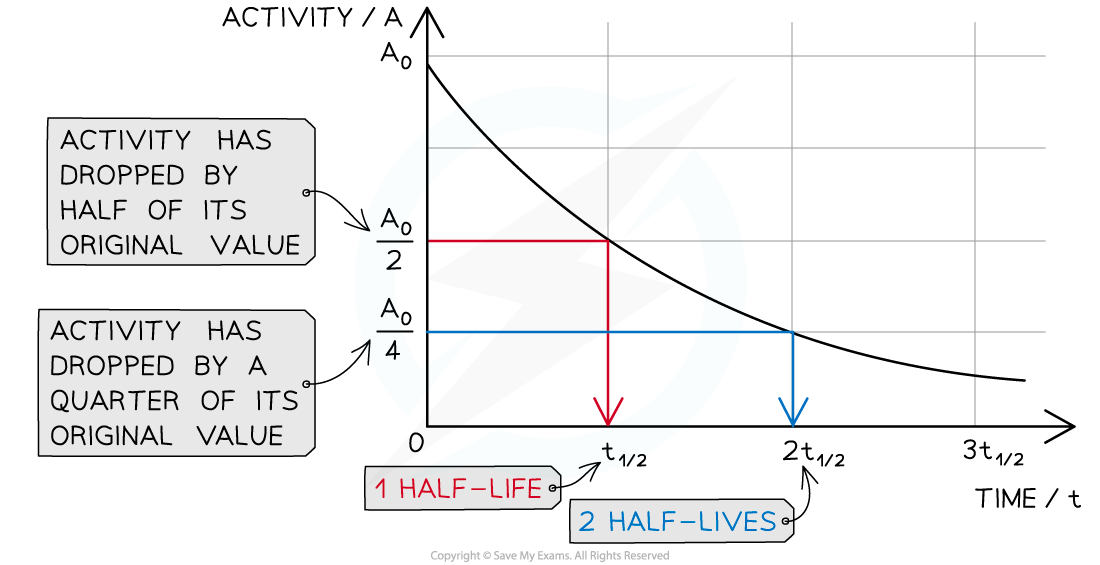• Compare found half-life for the radioactive sample with the known value

#### Evaluation

Systematic errors:

• The Geiger counter may suffer from an issue called “dead time”
• This is when multiple counts happen simultaneously within ~100 μs and the counter only registers one
• This is a more common problem in older detectors, so using a more modern Geiger counter should reduce this problem
• If this experiment is done manually, there will be uncertainty in the time
• This uncertainty in time intervals will mean variation in counting intervals, however, due to the 30-second interval and the nature of this experiment this is not easily accounted for
• It is best to discuss what these uncertainties could mean for the results of this experiment

Random errors:

• Radioactive decay is random, so repeat readings are vital in this experiment
• Measure the count over an appropriate time span such as 30 seconds
• A larger count helps reduce the statistical percentage uncertainty inherent in smaller readings
• This is because the percentage error is proportional to the inverse-square root of the count
• However, a shorter count will mean that more data points are available to help make an appropriate decay curve

#### Safety Considerations

• Reduce the exposure time by keeping it in a lead-lined box when not in use
• Handle with long tongs
• Avoid physical contact and handle the source with care
• Do not point the source at anyone and keep a large distance (as activity reduces by an inverse square law)
• Safety clothing such as a lab coat, gloves and goggles must be worn

#### Worked Example

A student measures the background radiation count in a laboratory and obtains the following readings:The student is trying to verify the half-life of a sample of Barium-137. He collects the following data: Use this data to determine the half-life of the radioactive samples.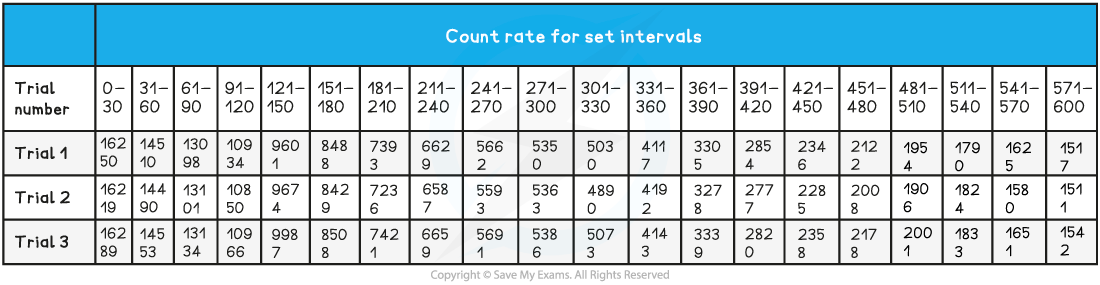Step 1: Determine a mean value of background radiationStep 2: Calculate C (corrected average count rate)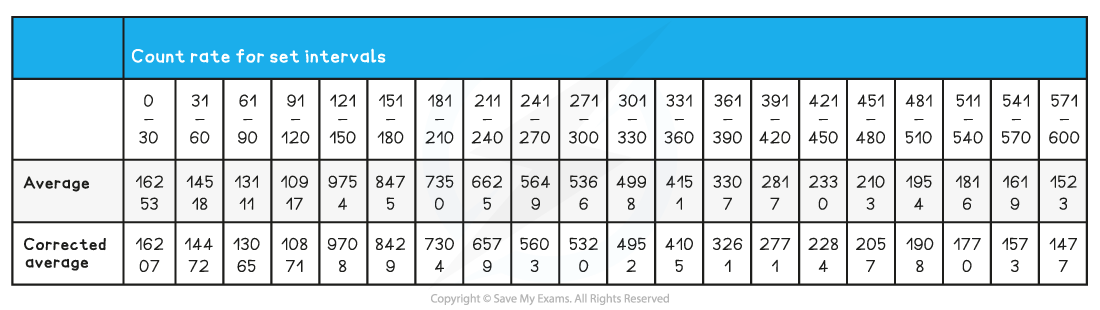Step 3: Plot a graph of C against (time in 30-sec intervals) and add a smooth-fitting curve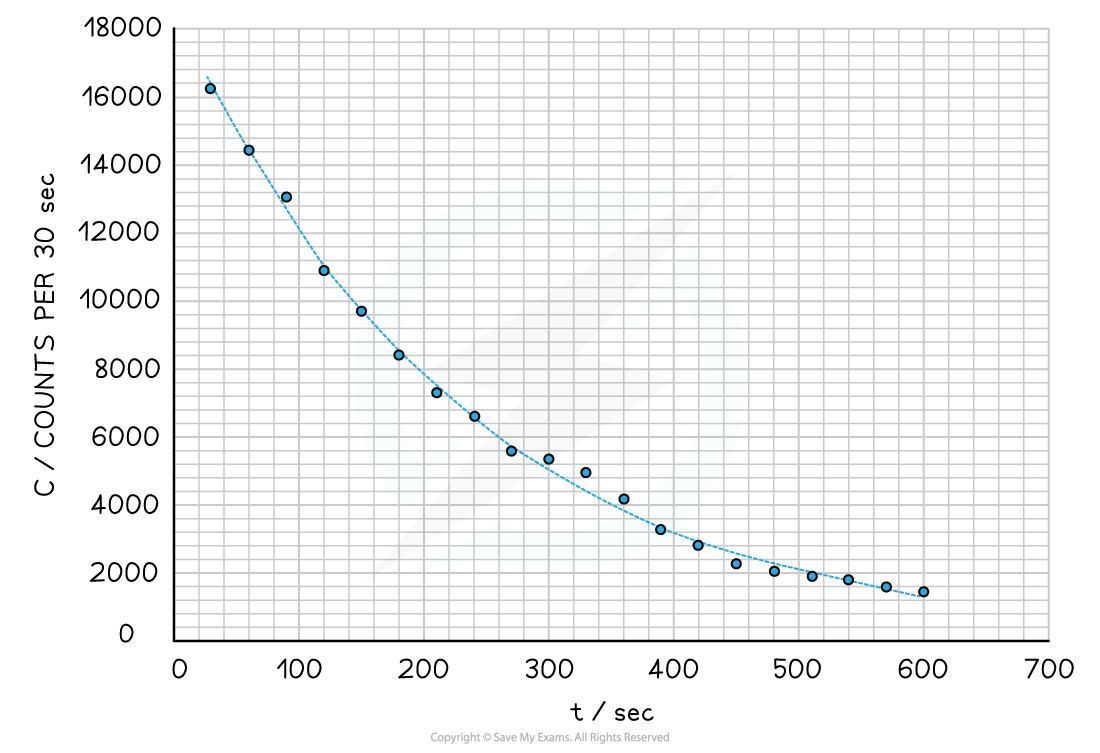Step 4: Map the first three half-lives use the curve

• The first half-life took approximately 160 seconds to occur
• The second half-life took approximately 150 seconds to be reached following the first half-life
• The third half-life took approximately 155 seconds to be reached following the second half-life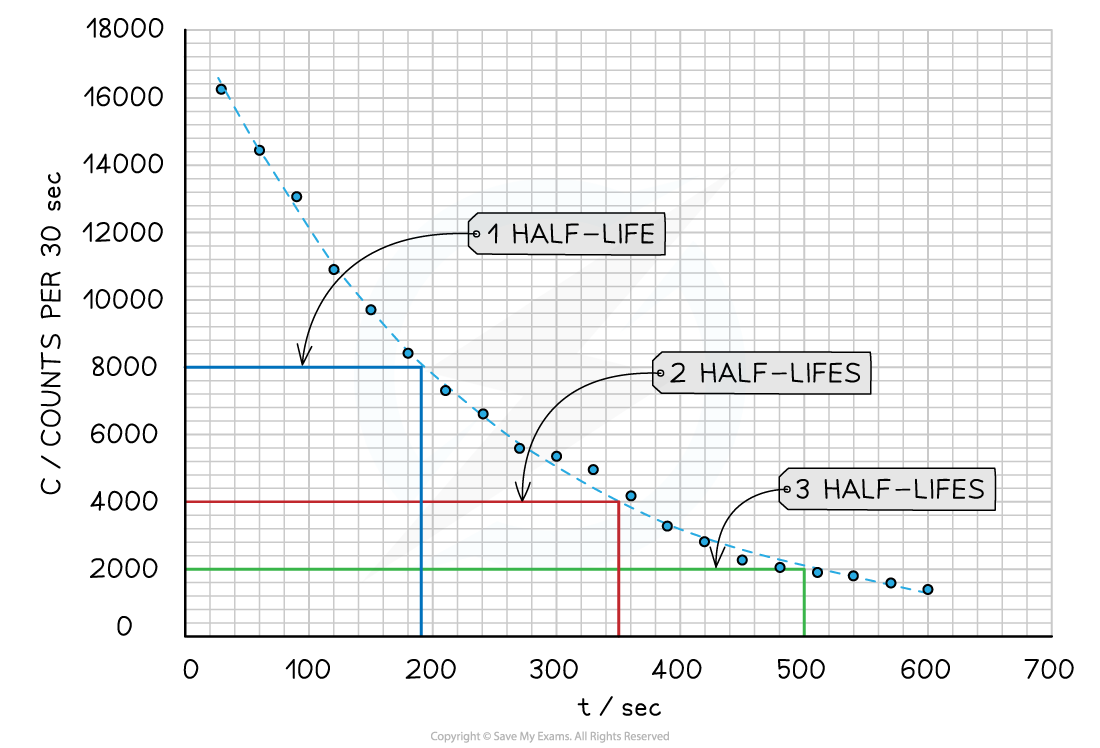Step 5: Average the half-lives

(160 + 150 + 155) ÷ 3 = 155 seconds

Step 6: State the final answer

• The final found answer was 155 seconds for the half-life of this sample data